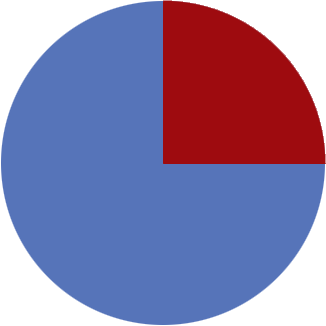SEARCH HOMEMath Central Quandaries & QueriesQuestion from sierra, a student: how do you figure the perimeter of a quarter circle that has a radius of 12Hi Sierra,

The perimeter (sometimes called the circumference) of a circle is $2 \times \pi \times r$ where $r$ is the radius and $\pi$ is approximately $3.1416.$ The perimeter of a quarter circle is composed of three parts, the curved part which has a length of one-quarter the perimeter of the circle and two straight parts, each of length $r.$The length of the perimeter of the quarter circle is the sum of the lengths of the three parts.

PennyMath Central is supported by the University of Regina and The Pacific Institute for the Mathematical Sciences.